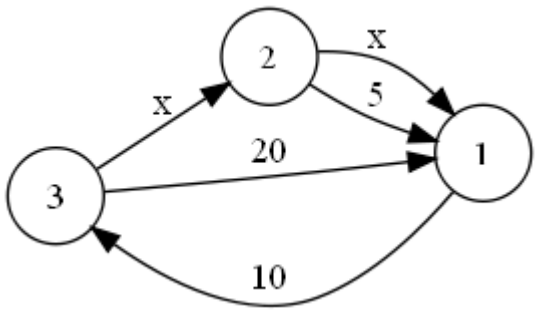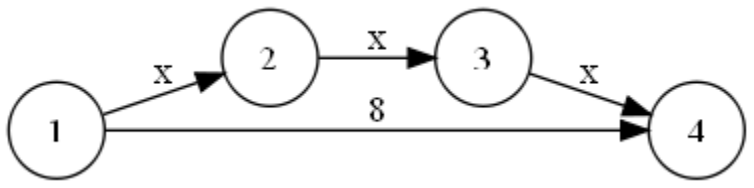시간 제한메모리 제한제출정답맞힌 사람정답 비율
5 초 64 MB90241527.273%

## 문제

In the distant future, food is transported between planets via one-way trade routes. Each route directly connects two planets and has a known transit time.

The traders' guild plans to add some new routes utilizing a recently discovered technology – hyperspace travel. Travelling via hyperspace is also one-directional. Since it is still experimental, hyperspace travel time is not yet known, however it is known not to depend on distances between planets, so each hyperspace route will take an equal amount of time to traverse.

The picture below shows an example of three interconnected planets with transit times shown. The planets are labelled with positive integers, and the hyperspace travel time is denoted by “x” (the picture corresponds to the second example input):Transit time is measured in days and is always a positive integer.

The traders' guild wishes to analyze the consequences of introducing the new routes: for some two planets A and B, they want to know what are all the possible values of the shortest path total transit time from A to B, for all possible values of x. For example, in the situaton above, shortest path travel from planet 2 to planet 1 could take 5 (if x ≥ 5), 4, 3, 2, or 1 day (if x < 5).

## 입력

The first line of input contains the two integers P and R, the number of planets and the number of routes, respectively (1 ≤ P ≤ 500, 0 ≤ R ≤ 10 000).

Each of the following R lines contains two integers C and D, the planet labels (1 ≤ C, D ≤ P, C ≠ D), and T, the travel time from C to D. For conventional routes, T is an integer (1 ≤ T ≤ 1 000 000), and for hyperspace routes, T is the character “x”. Multiple lines can exist between the same two planets.

The following line contains the integer Q, the number of queries (1 ≤ Q ≤ 10).

Each of the following Q lines contains two integers A and B, the planet labels (A ≠ B) representing a query by the traders' guild: “what are the possible values of shortest path transit time from A to B?”.

## 출력

The output must contain Q rows, one per query.

Each row must contain two integers: the number of different values and their sum. If the number of different values is unbounded, output only "inf" in that row. If there is no path from A to B, the number of different values and their sum is 0.

## 예제 입력 1

4 4
1 2 x
2 3 x
3 4 x
1 4 8
3
2 1
1 3
1 4


## 예제 출력 1

0 0
inf
3 17


## 예제 입력 2

3 5
3 2 x
2 1 x
2 1 5
1 3 10
3 1 20
6
1 2
2 3
3 1
2 1
3 2
1 3


## 예제 출력 2

inf
5 65
15 185
5 15
inf
1 10


## 힌트

Clarification of the first example:

1. There is no possible path from 2 to 1.
2. For any positive integer x, the shortest path from 1 to 3 takes 2x time, so the solution is "inf".
3. The shortest path from 1 to 4 can take 3 (for x = 1), 6 (for x = 2), or 8 (for x >= 3) time. 3+6+8=17